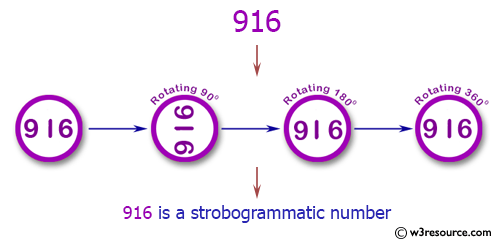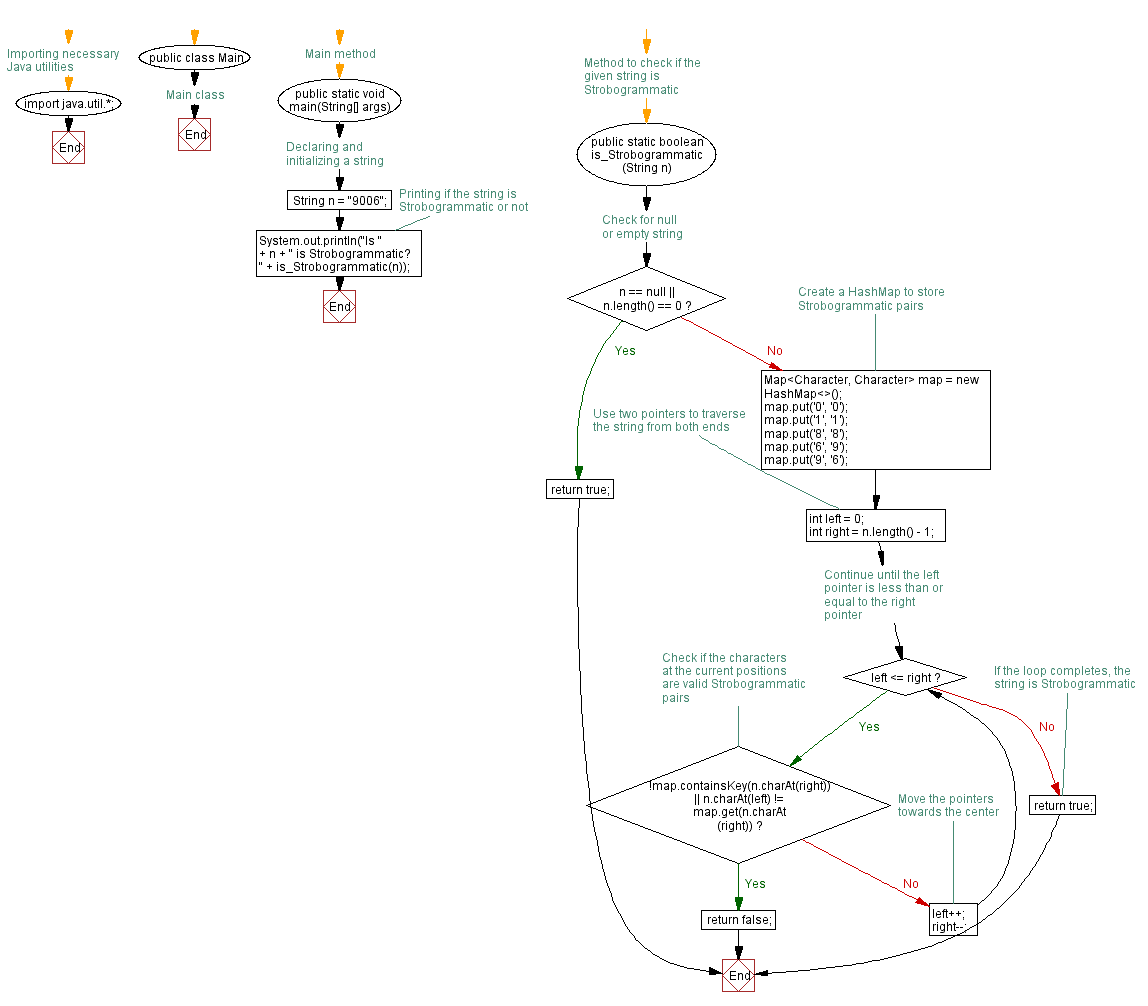﻿ Java programming exercises: Check if a number is a strobogrammatic number - w3resource# Java Exercises: Check if a number is a strobogrammatic number

## Java Basic: Exercise-186 with Solution

Write a Java program to check if a number is a strobogrammatic number. The number is represented as a string.

According to Wikipedia "A strobogrammatic number is a number whose numeral is rotationally symmetric, so that it appears the same when rotated 180 degrees. In other words, the numeral looks the same right-side up and upside down (e.g., 69, 96, 1001). A strobogrammatic prime is a strobogrammatic number that is also a prime number, i.e., a number that is only divisible by one and itself (e.g., 11). It is a type of ambigram, words and numbers that retain their meaning when viewed from a different perspective, such as palindromes."
The first few strobogrammatic numbers are:
0, 1, 8, 11, 69, 88, 96, 101, 111, 181, 609, 619, 689, 808, 818, 888, 906, 916, 986, 1001, 1111, 1691, 1881, 1961, 6009, 6119, 6699, 6889, 6969, 8008, 8118, 8698, 8888, 8968, 9006, 9116, 9696, 9886, 9966, ...

Pictorial Presentation:Sample Solution:

Java Code:

``````import java.util.*;
public class Solution {
public static void main(String[] args) {
String n = "9006";
System.out.println("Is " + n + " is Strobogrammatic? " + is_Strobogrammatic(n));
}

public static boolean is_Strobogrammatic(String n) {
if (n == null || n.length() == 0) {
return true;
}
HashMap < Character, Character > map = new HashMap < Character, Character > ();
map.put('0', '0');
map.put('1', '1');
map.put('8', '8');
map.put('6', '9');
map.put('9', '6');
int left = 0;
int right = n.length() - 1;
while (left <= right) {
if (!map.containsKey(n.charAt(right)) || n.charAt(left) != map.get(n.charAt(right))) {
return false;
}
left++;
right--;
}
return true;
}
}
```
```

Sample Output:

```Is 9006 is Strobogrammatic? true
```

Flowchart:Java Code Editor:

Company:

What is the difficulty level of this exercise?

﻿

## Java: Tips of the Day

Parsing dates:

```import java.io.*;
import java.util.*;
import java.text.*;

String s = "2001/09/23 14:39";

SimpleDateFormat formatter = new SimpleDateFormat ("yyyy/MM/dd H:mm");
Date d = formatter.parse(s, new ParsePosition(0));
```